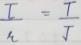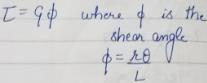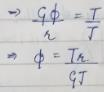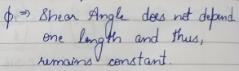Courses

# Torsion Of Shafts - 2

## 10 Questions MCQ Test Mock Test Series for Civil Engineering (CE) GATE 2020 | Torsion Of Shafts - 2

Description
This mock test of Torsion Of Shafts - 2 for Civil Engineering (CE) helps you for every Civil Engineering (CE) entrance exam. This contains 10 Multiple Choice Questions for Civil Engineering (CE) Torsion Of Shafts - 2 (mcq) to study with solutions a complete question bank. The solved questions answers in this Torsion Of Shafts - 2 quiz give you a good mix of easy questions and tough questions. Civil Engineering (CE) students definitely take this Torsion Of Shafts - 2 exercise for a better result in the exam. You can find other Torsion Of Shafts - 2 extra questions, long questions & short questions for Civil Engineering (CE) on EduRev as well by searching above.
QUESTION: 1

### A shaft turns at 150 rpm under a torque of 150 Nm. Power transmitted is

Solution:

Power transmitted is given by,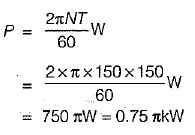QUESTION: 2

### The outside diameter of a hollow shaft is twice its inside diameter. The ratio of its torque carrying capacity to that a solid shaft of the same material and the same outside diameter is

Solution:

The torque carrying capacity Tis given by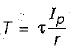Let outside diameter of hollow and solid shaft = 2d
∴ Inside diameter of hollow shaft=  d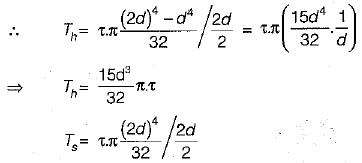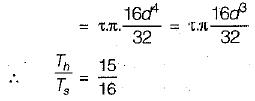QUESTION: 3

### A round shaft of diameter ‘d and length T fixed at both ends A and B is subjected to a twisting moment T at C, at a distance 114 from A. The torsional stresses in the parts AC and CB will be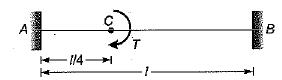Solution: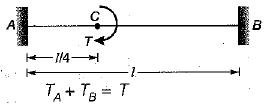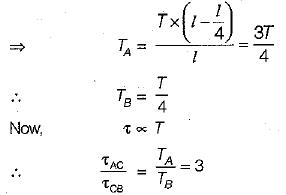QUESTION: 4

A solid shaft of diameter D carries a twisting moment that develops maximum shear stress τ. if the shaft is replaced by a hollow one of outside diameter ‘D’ and inside diameter D/2, then the maximum shear stress will be

Solution: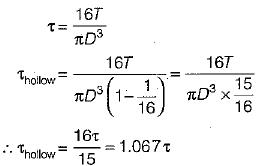QUESTION: 5

In a rectangular shaft subjected to torsion, the maximum shear occurs at

Solution:
QUESTION: 6

The ratio of the moment of resistance of a solid circular shaft of diameter D and a hollow shaft (external diameter D and internal diameter d) is given by

Solution:

As we know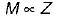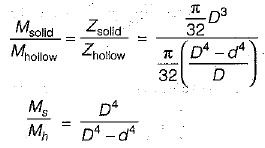QUESTION: 7

If a shaft of diameter d is subjected to a torque Tand a bending moment M, the maximum shear stress is

Solution:
QUESTION: 8

The diameter of shaft B is twice that of shaft A Both shafts have the same length and are of the same material, if both are subjected to same torque, then the ratio of the angle of twist of shaft' A to that of shaft B will be

Solution: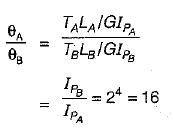QUESTION: 9

if a shaft is rotating at N revolution per second with an applied torque T kg-m, the\power transmitted by the shaft in watt is

Solution:

Power transmitted by the shaft is given by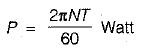if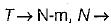Revolutions per minute
When T is in kgm
and N is in revolutions per second,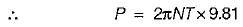QUESTION: 10

A shaft of diameter 'd' and length L is subjected to twisting moment T, shear angle developed in shaft is 0.001 rad. Now the length of the shaft is doubled, but the diameter and torque remain the same what will be the shear angle?

Solution: